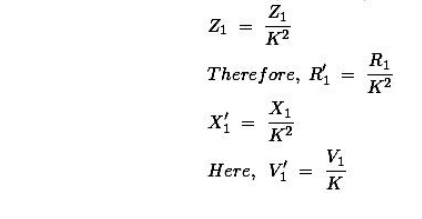Home | | Electrical Engineering and Instrumentation | Equivalent Circuit of Transformer

# Equivalent Circuit of Transformer

1. Equivalent Circuit of Transformer Referred to Primary 2. Approximate Equivalent Circuit of Transformer 3. Equivalent Circuit of Transformer Referred to Secondary

Equivalent Circuit of Transformer

Equivalent impedance of transformer is essential to be calculated because the electrical power transformer is an electrical power system equipment for estimating different parameters of electrical power system which may be required to calculate total internal impedance of an electrical power transformer, viewing from primary side or secondary side as per requirement. This calculation requires equivalent circuit of transformer referred to primary or equivalent circuit of transformer referred to secondary sides respectively. Percentage impedance is alsovery essential parameter of transformer. Special attention is to be given to this parameter during installing a transformer in an existing electrical power system. Percentage impedance of different power transformers should be properly matched during parallel operation of power transformers. The percentage impedance can be derived from equivalent impedance of transformer so, it can be said that equivalent circuit of transformer is also required during calculation of % impedance.

## 1. Equivalent Circuit of Transformer Referred to Primary

For drawing equivalent circuit of transformer referred to primary, first we have to establish general equivalent circuit of transformer then; we will modify it for referring from primary side. For doing this, first we need to recall the complete vector diagram of a transformer as shown in figure 2.7.Let us consider the transformation ratio be,In figure 2.7, the applied voltage to the primary is V1 and voltage across the primary winding is E1. Total current supplied to primary is I1. So the voltage V1 applied to the primary is partly dropped by I1Z1 or I1R1 + j.I1X1 before it appears across primary winding. The voltage appeared across winding is countered by primary induced emf E1.From the vector diagram above, it is found that the total primary current I1 has two components, one is no - load component Io and the other is load component I2′. As this primary current has two a component or branches, so there must be a parallel path with primary winding of transformer. This parallel path o f current is known as excitation branch of equivalent circuit of transformer. The resistive and reactive branches of the excitation circuit can be represented as,The equivalent circuit for that equation can be drawn as below,Again I2′.N1 = I2.N2From above equation, secondary impedance of transformer referred to primary is,## 2. Approximate Equivalent Circuit of Transformer

Since Io is very small compared to I1, it is less than 5% of full load primary c urrent, Io changes the voltage drop insignificantly. Hence, it is good approximation to ignore the excitation circuit in approximate equivalent circuit of transformer. The winding resistance and reactance being in series can now be combined int o equivalent resistance and reactance of transformer, referred to any particular side. In this case it is side 1 or primary side.## 3. Equivalent Circuit of Transformer Referred to Secondary

In similar way, approximate e quivalent circuit of transformer referred to secondary can be drawn. Where equivalent impedance of transformer referred to secondary, can b e derived as,Study Material, Lecturing Notes, Assignment, Reference, Wiki description explanation, brief detail
Electrical Engineering and Instrumentation : Transformer : Equivalent Circuit of Transformer |